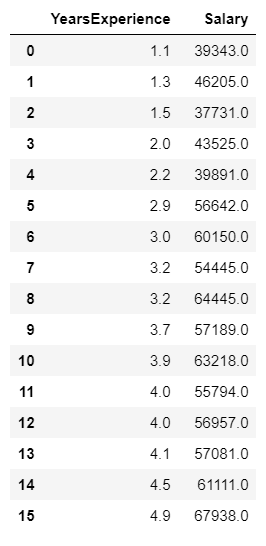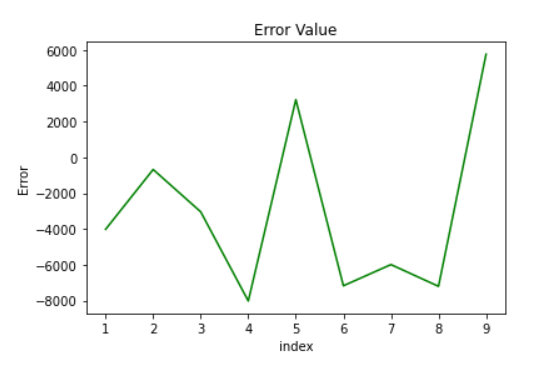Search

# Linear Regression : A practical approach with python.

If you are looking for a quick solution, How to write a code to implement or analyze linear regression, you are in the right place.

Before jumping to the practical approach, let me ask you the question, What is the linear regression, and How many types of it?

Question: What is a linear regression?

Answer: In statistics, linear regression is a linear approach to modeling the relationship between a scalar response (or dependent variable) and one or more explanatory variables (or independent variables).

Question: How many types of Liner regression?

Answer: There are two types of linear regression.

1. Simple linear regression.

2. Multiple linear regression.

We will see How to implement simple linear regression.

Now we take a simple dataset to find the linear regression between two variables.This is the simple dataset where one variable represents how many years experience the employee has and how much salary he/she is earning according to the experience.

We will first load the dataset in python using panda and then we will plot the data to scatter plot.

Then we will apply variables to X and Y-axis.

Then we will Import the Linear Regression model from scikit learn.

After that, we will find the predicted and an error value.

The final step is to find the intercept and coefficient of the line.

Fig1: Dataset

```# import all the lib
import numpy as np
import pandas as pd
import matplotlib.pyplot as plt
import seaborn as sns```

```#read dataset using pandas
set.```

Plotting the scatter plot using the matplotlib library.

```plt.figure(figsize=(12,6))
sns.pairplot(data,x_vars=['YearsExperience'],y_vars=['Salary'],size=7,kind='scatter')
plt.xlabel('Years')
plt.ylabel('Salary')
plt.title('Salary Prediction')
plt.show()```

You will able to see the scatter plot graph below.Fig1: Scatter Plot of Salary vs Years of Experience.

Applying Years of experience to X-Axis.

```X = data['YearsExperience']

Output: 0 1.1 1 1.3 2 1.5 3 2.0 4 2.2 Name: YearsExperience, dtype: float64

Applying Salary to Y-Axis.

```y = data['Salary']

Output: 0 39343.0 1 46205.0 2 37731.0 3 43525.0 4 39891.0 Name: Salary, dtype: float64

```# Import Segregating data from scikit learn
from sklearn.model_selection import train_test_split```

Now we will train the dataset.

`X_train,X_test,y_train,y_test = train_test_split(X,y,train_size=0.7,random_state=100)`

Creating new axis for x column for the training dataset and testing dataset.

```# Create new axis for x column
X_train = X_train[:,np.newaxis]
X_test = X_test[:,np.newaxis]```
```# Importing Linear Regression model from scikit learn
from sklearn.linear_model import LinearRegression```

Apply Linear regression to the training dataset.

```# Fitting the model
lr = LinearRegression()
lr.fit(X_train,y_train)```

Output: LinearRegression()

Now from a trained linear regression model predicting the salary using test data.

```# Predicting the Salary for the Test values
y_pred = lr.predict(X_test)```

```# Plotting the actual and predicted values
c = [i for i in range (1,len(y_test)+1,1)]
plt.plot(c,y_test,color='r',linestyle='-')
plt.plot(c,y_pred,color='b',linestyle='-')
plt.xlabel('Salary')
plt.ylabel('index')
plt.title('Prediction')
plt.show()```Fig : 3 Prediction of salary using the test dataset

```# plotting the error
c = [i for i in range(1,len(y_test)+1,1)]
plt.plot(c,y_test-y_pred,color='green',linestyle='-')
plt.xlabel('index')
plt.ylabel('Error')
plt.title('Error Value')
plt.show()```Fig4: Error value of the test dataset.

Finding the intercept and coefficient.

```# Intercept and coeff of the line
print('Intercept of the model:',lr.intercept_)
print('Coefficient of the line:',lr.coef_)```

Output: Intercept of the model: 25202.887786154883 Coefficient of the line: [9731.20383825]

Conclusion:

Question: Why we are finding intercept?

Answer: The intercept (often labeled as constant) is the point where the function crosses the y-axis. In some analyses, the regression model only becomes significant when we remove the intercept, and the regression line reduces to Y = bX + error.